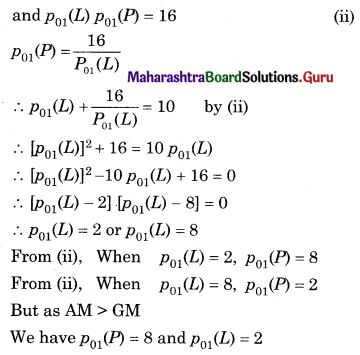# Maharashtra Board 12th Commerce Maths Solutions Chapter 5 Index Numbers Ex 5.2

Balbharati Maharashtra State Board 12th Commerce Maths Solution Book Pdf Chapter 5 Index Numbers Ex 5.2 Questions and Answers.

## Maharashtra State Board 12th Commerce Maths Solutions Chapter 5 Index Numbers Ex 5.2

Calculate Laspeyres, Paasche’s, Dorbish-Bowely’s, and Marshall-Edegworth’s Price Index Numbers in Problems 1 and 2.

Question 1.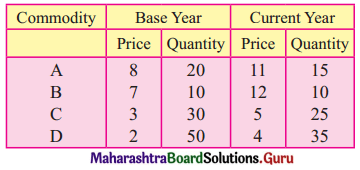Solution: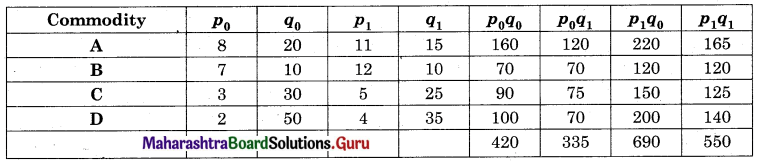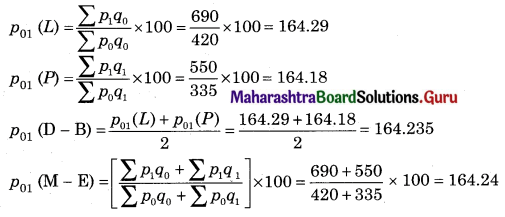Question 2.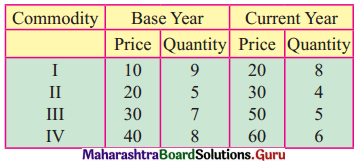Solution: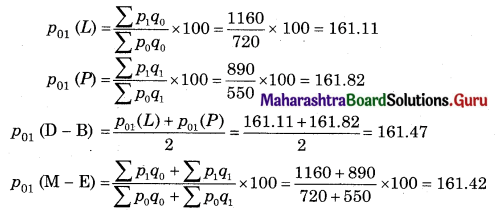Calculate Walsh’s Price Index Number in Problems 3 and 4.

Question 3.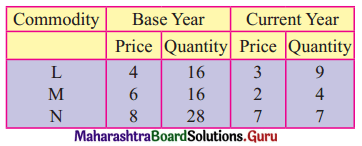Solution: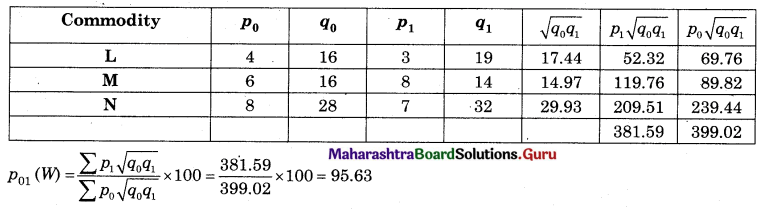Question 4.Solution: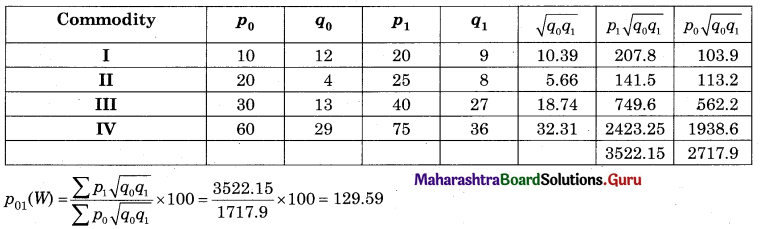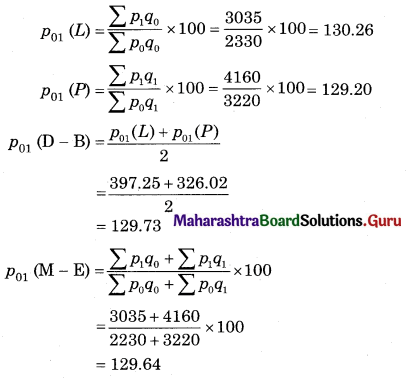Question 5.
If p01(L) = 90, and p01(P) = 40, find p01(D – B) and p01(F)
Solution: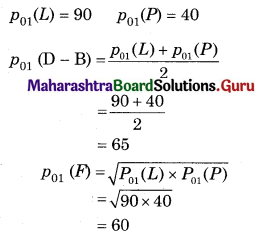Question 6.
If Σp0q0 = 140, Σp0q1 = 200, Σp1q0 = 350, Σp1q1 = 460, find Laspeyre’s Paasche’s Dorbish-Bowley’s and Marshall- Edgeworth’s Price Index Numbers.
Solution:Question 7.
Given that Laspeyre’s and Dorbish-Bowley’s Price Index Numbers are 160.32 and 164.18 respectively. Find Paasche’s Price Index Number.
Solution: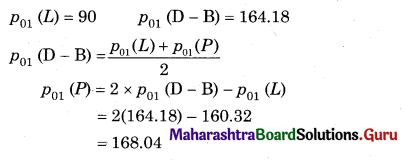Question 8.
Given that Σp0q0 = 220, Σp0q1 = 380, Σp1q1 = 350 is Marshall-Edgeworth’s Price Index Number is 150, find Laspeyre’s Price Index Number.
Solution:Question 9.
Find x in the following table if Laspeyres and Paasche’s Price Index Numbers are equal.Solution: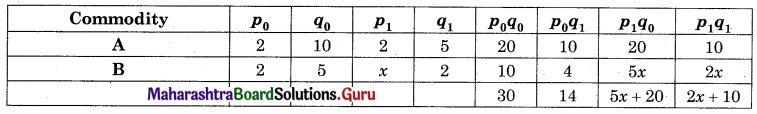Question 10.
If Laspeyre’s Price Index Number is four times Paasche’s Price Index Number, then find the relation between Dorbish-Bowley’s and Fisher’s Price Index Numbers.
Solution:Question 11.
If Dorbish-Bowley’s and Fisher’s Price Index Numbers are 5 and 4, respectively, then find Laspeyres and Paasche’s Price Index Numbers.
Solution: